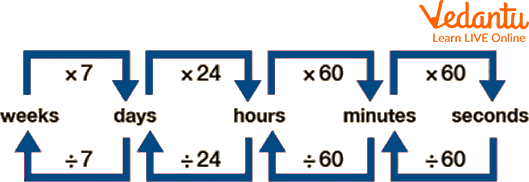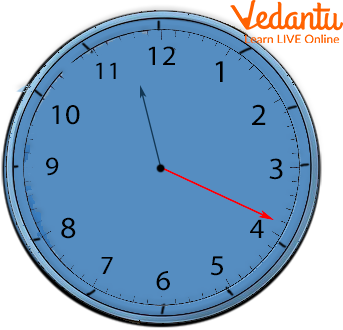Courses
Courses for Kids
Free study material
Free LIVE classes
More

# Conversion of TimeLIVE
Join Vedantu’s FREE Mastercalss

## Introduction to the Conversion of Time

Time is how we measure the duration of an activity, a task, or maybe a season. The tool used for ages for this purpose is a clock that has two main hands, i.e., the minute hand and hour hand. The minute hand goes round the clock every 60 minutes to complete an hour.

Time can be measured in some units, including seconds, minutes, hours, days, weeks, months, and years. 1 hour =60 minutes. It needs 12 hours to complete one full circle of the clock. A day has a total of 24 hours, and the hour hand goes round the clock twice. 1 day =24 hours. And 7 such days form a week. A year has a total of 12 months.

Here, the conversion of time is explained in detail. The most important and tricky question of how many seconds in a year is also cleared below in the article.

## Leap Year

• Leap years are those years in which the entire year has 366 days. Earth requires one year to make one revolution around the sun.

• But the exact time taken for that revolution is $365 \frac{1}{4}$ days or 365 days and 6 hours. These six hours don't get counted every year and are added every fourth year.

• That is why every fourth year has an extra day adding up those 6 years making it a total of 366 days.

• The extra day is added to the month of February and makes it 29 days.

## Conversion Table/ Time Unit Conversion Chart

Time can be grouped into various units. The unit conversion chart table is given below as follows:

 1 minute 60 seconds 1 hour 60 minutes 1 day 24 hours 1 week 7 days 1 year (days) 365 days 1 year (months) 12 months 1 year (weeks) 52 weeks

## How Many Seconds in a Year?

How many seconds are in a year? The answer is cleared below with the help of the conversion table given above. 31,536,000 seconds are present within a year which is a total of 365 days.

## Time Conversion Chart

Time conversion chart can be used to understand the time very minutely and accurately.Time Conversion Chart.

## Conversion of Days into Hours

So let's take an example of 7 days and let us see how many hours are packed into 7 days. The conversion rule is that 1 day is equal to 24 hours.

Therefore, let us multiply 7 by 24

$7 \times 24 = 168$ hours

Now if we had to convert days and hours into hours altogether.

We have to convert the days into hours and add the remaining hours to the converted value.

For example, convert 7 days 5 hours to hours.

We begin with converting the days which are 7 into hours, i.e. $7 \times 24 = 168$ hours

Now, add the remaining hours, i.e. $168 + 5 = 173$ hours.

## Conversion of Hours into Minutes

We know that 1 hour $=60$ minutes

So if we had to calculate how many minutes make hours,

We multiply hours by 60

hours $=5 \times 60 = 300$ minutes

Now, let us convert hours and minutes into minutes

Let's take the example of 8 hours and 30 minutes

8 hours 30 minutes

$=(8 \times 60)+30 \text { minutes } = 480 + 30 \text { minutes } = 510 \text { minutes }$

## Conversion of Minutes into Seconds

We know that, 1 minute $=60$ seconds

So if we had to calculate how many seconds make 20 minutes,

We multiply 20 with 60,

$20 \times 60$ seconds $=1200$ seconds

Now, let us convert minutes and seconds into seconds for 40 minutes and 100 seconds

We convert 40 minutes into seconds,

$=40 \times 60$ seconds $=2400$ seconds

$=2400+100 \text { seconds }=2500 \text { seconds }$Cloth with Hour, Minute and Second Hand.

Below are some of the examples and some practice problems on the conversion of time.

## Solved Examples

Q1. Convert 30 minutes into seconds.

Ans: We know that 1 minute = 60 seconds,

So if we had to calculate how many seconds make 30 minutes,

We multiplied 30 by 60,

Therefore, the total number of seconds in 30 minutes is 1800 seconds.

Q2. Convert 3 days 12 hours into hours.

Ans: The conversion rule is that 1 day equals 24 hours. Therefore, we need to multiply 3 with 24 and add 12 to that value.

3 x 24 = 72 hours.

To obtain total hours in 3 days and 12 hours, 72 + 12 = 84 hours.

Q3. Convert 9 hours and 10 minutes into minutes.

Ans: The rule for the conversion of hours into minutes is that each hour has a total of 60 minutes.

Therefore, we multiply 9 by 60, and after that, add 10 minutes.

9 x 60 minutes = 540 minutes

540 + 10 minutes = 550 minutes.

## Practice Questions

Q 1. Convert 16 minutes into seconds.

Ans: 960 seconds

Q 2. Convert 5 days 6 hours into hours.

Ans: 126 hours

Q 3. Convert 2 hours 25 minutes into minutes.

Ans: 145 minutes

## Summary

Time is the unit by which we define the length of a work, an activity, or a season. Since ancient times, a clock with two primary hands—the minute hand and the hour hand—has been used for this purpose. Every hour and a half, the minute hand completes a full rotation of the clock. Leap year has 366 days, and it comes each fourth year.

The conversion table of time is a necessary tool for various mathematical calculations. Conversion of time either involves multiplication or division to change the unit. This means that if you take a larger unit and convert it to a smaller unit, you must multiply. But if you take a smaller unit and convert it to a larger one, you must divide. There are three main units for time, i.e. hours, minutes, and seconds. Time relation is: 60 seconds = 1 minute, 60 minutes = 1 hour, 24 hours = 1 day, 7 days = 1 week, 12 months = 1 year.

Last updated date: 24th Sep 2023
Total views: 108.9k
Views today: 1.08k

## FAQs on Conversion of Time

1. What is a leap year?

Leap years are those years in which the entire year has 366 days. Earth requires one year to make one revolution around the sun. But the exact time taken for that revolution is $365 \frac{1}{4}$ days or 365 days and 6 hours. These six hours don't get counted every year and are added every fourth year. That is why every fourth year has an extra day adding up those 6 years, making it a total of 366 days.

2. What is the importance of conversion in general?

We need to convert between units to ensure accuracy and prevent measurement misinterpretation. For example, we do not measure a pencil's length in kilometres. In this situation, one must convert from kilometres (km) to centimetres (cm).

3. What does time signify in Mathematics?

Time can be described in Mathematics as an ongoing and continuous series of events that occur one after another, from the past through the present and into the future. The duration of events or the gaps between them can be measured, compared, or even ordered using time.